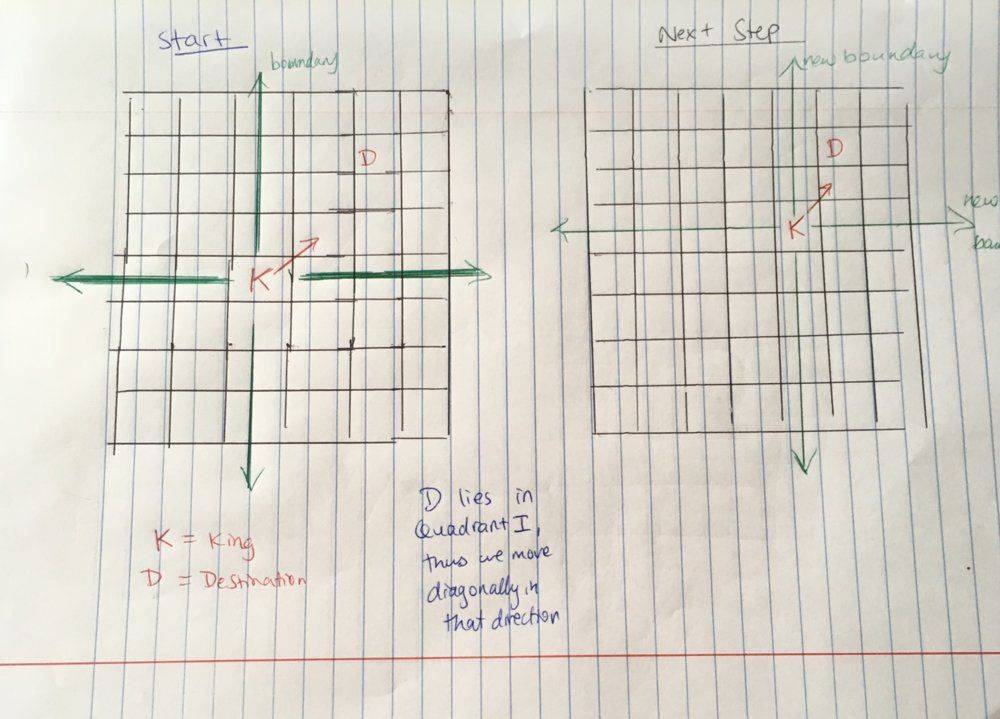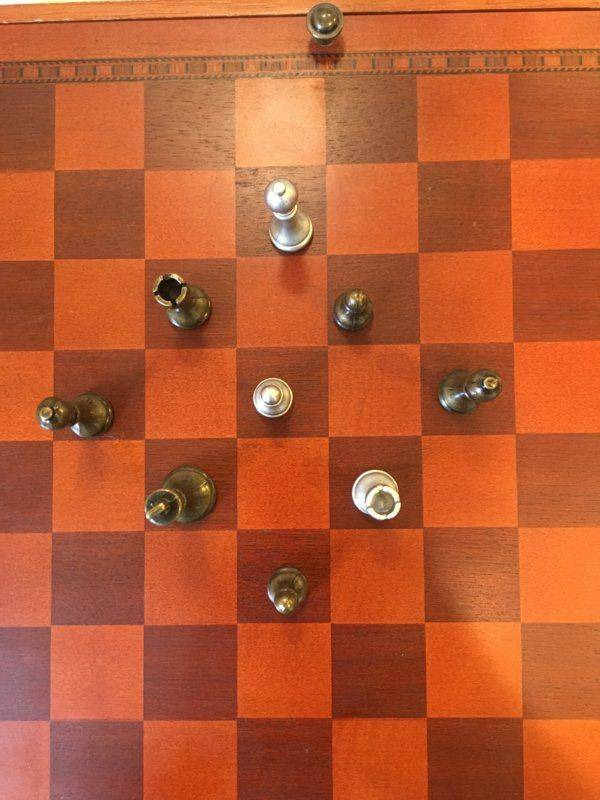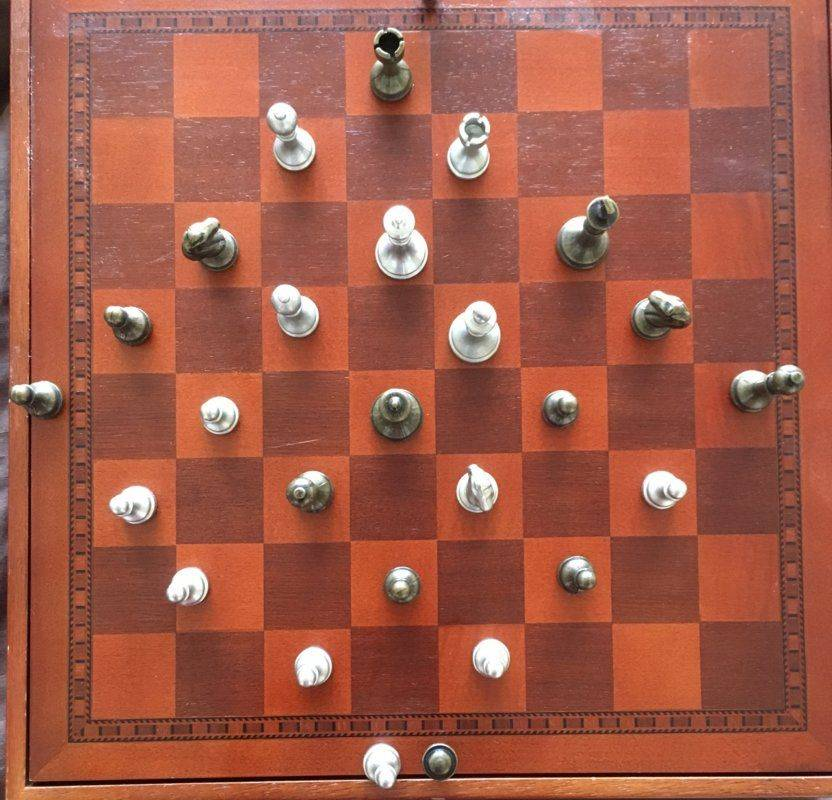# Math Challenge - April 2019

• Challenge
Gold Member
Let us fix our king at some arbitrary origin, and then let us draw lines that extend in the four 'cardinal' directions around the king. Here is a more qualitative description of the method first:
1. Set up boundary lines for your king emanating from his current position
2. For a given destination, evaluate whether it lies on any of the 4 boundary lines
- If it lies on a boundary line, then move in that respective direction and then go back to step 2
3. If not, see which quadrant the destination is in and move diagonally 1 move in that direction - then go back to one and repeat

I see a good effort here but I can't visualize it in the exact way needed.

Let us define a cartesian coordinate system such that the centre of each square is defined by a pair of integer coordinates ##(x,y)## and our King will start at ##(0,0)## .

For a given destination ##(a,b)## , let us assume that ##a > b## . We will end up moving diagonally b times and then vertically ##a - b## times. Therefore, for destination ##(a,b)## , we will only move ##|a| - |b|+ |b| = |a|## times.

This makes sense following on from part (a). Thinking about the possible squares (that the king can land after n moves) as an area of a square ##(2n + 1)^2## as written by fbs7 for the general n. For us to reach a square in the minimum number of moves ##(n)## , we need ##n## such that it is the first square to include our destination point. This will only happen if n = number of ##\textbf{n = number of moves = max(|a|,|b|)}## for all n.

I don't ask for a rigorous way of describing the minimum number of moves required to reach a destination. I just ask for the optimal strategy for this, described as a number of steps. So, moving in the diagonal direction as you say, is the first step. Now, from what you write I see that you're on the right way for the next step but how can we describe it in simple words i.e. next, what is the move in order to reach the destination, independently of whether it is on the diagonal or not?

Master1022
I see a good effort here but I can't visualize it in the exact way needed.

Thank you for your response. I have attached a quick sketch to try to help visualise my thinking.Edit: Please let me know if this is not legible

Gold Member
Here is my attempt at Highschool problem 1(c)...

For even ##n \gt 0## the number of squares is not correct. Check it again.

Gold Member
I have attached a quick sketch to try to help visualise my thinking.

Yes. So, again to my question: You're moving diagonally and if the destination is not on the diagonal, what is the next move?

Master1022
For even ##n \gt 0## the number of squares is not correct. Check it again.
Thank you for your response. Is the answer not $(n+1)^2$, which is equivalent to what I have written? I thought it would be this as it will be the total number of squares of the same colour as the starting square within our region of furthest possible squares.

Master1022
Yes. So, again to my question: You're moving diagonally and if the destination is not on the diagonal, what is the next move?
Thanks for your response. Maybe my steps weren't clear enough. However, to address your question, do we just move in one cardinal direction that will most quickly put us on a similar diagonal with the destination? However, isn't this method the same as the one as I outlined, with the steps just reversed?

Last edited:
Gold Member
However, to address your question, do we just move in one cardinal direction that will most quickly put us on a similar diagonal with the destination? However, isn't this method the same as the one as I outlined, with the steps just reversed?

Let's take it in a simple way. We are moving along a diagonal - whichever it is. If we meet our target (=destination) we're done. If not , there is a simple way to state what you do according to where is the destination relatively to the point on the diagonal that you are. That's all I ask. The way you describe it is as taking another diagonal etc. Is this the optimal way?

Gold Member
Is the answer not ##(n+1)^2## , which is equivalent to what I have written? I thought it would be this as it will be the total number of squares of the same colour as the starting square within our region of furthest possible squares.

each new 'layer' will have ##4n## squares of possible destinations.

For instance take ##n = 2##. Then what you write means that starting from, say, a black square, the possible squares of the new layer, as you call it, will be ##8##. Now I ask, is this premise correct?

EDIT: As a hint, if you take the next positive even integer i.e. ##n = 4## then what I ask above may be more evident.

Last edited:
Master1022

For instance take ##n = 2##. Then what you write means that starting from, say, a black square, the possible squares of the new layer, as you call it, will be ##8##. Now I ask, is this premise correct?

EDIT: As a hint, if you take the next positive even integer i.e. ##n = 4## then what I ask above may be more evident.

Thank you for your response. This is what I found for n = 2 and n = 4. Are you saying that I am missing some squares?The outer layers seem to have 4n squares in them, so I will keep looking

Gold Member
@Master1022 as I check again High School problem ##1 c## I see that you mean that the total sum is ##1## plus a new layer (=possible positions) each time and the series is on even numbers, so you finally mean ##(n + 1)^2## but the way is written is more cumbersome than it needs to. So, what is the difference from ##n## being odd?

Master1022
@Master1022 as I check again High School problem ##1 c## I see that you mean that the total sum is ##1## plus a new layer (=possible positions) each time and the series is on even numbers, so you finally mean ##(n + 1)^2## but the way is written is more cumbersome than it needs to. So, what is the difference from ##n## being odd?
I realized after posting that they were the same, but not when I was working through the mathematics.

•QuantumQuest
Master1022
Let's take it in a simple way. We are moving along a diagonal - whichever it is. If we meet our target (=destination) we're done. If not , there is a simple way to state what you do according to where is the destination relatively to the point on the diagonal that you are. That's all I ask. The way you describe it is as taking another diagonal etc. Is this the optimal way?
Thank you for your response. I fail to see how my method doesn't get a king/piece to the destination square in an optimal number of moves. In response to your suggestion, I will keep thinking. At first, I thought about closest approach (i.e. you move diagonally until the direction between you and the destination is perpendicular to the diagonal you move on, but that only works if destination square is of the same colour as the diagonal). Would you be able to explain what is redundant in my method which is basically "move diagonally towards the destination until you are below/above/left/right it, then move straight towards it"?

Gold Member
I realized after posting that they were the same, but not when I was working through the mathematics.

So, in both cases it is ##(n + 1)^2## squares and you get the credit for it.

As a simpler way to analyze and state the whole thing, you can say that starting from a certain square whether white or black, for each new ##n##, we take into account all the squares of the same color (##n## even) or the opposite color (##n## odd) that are on the "border" - created by these squares, plus the squares of the same color (with the ones on the border) inside it.

•